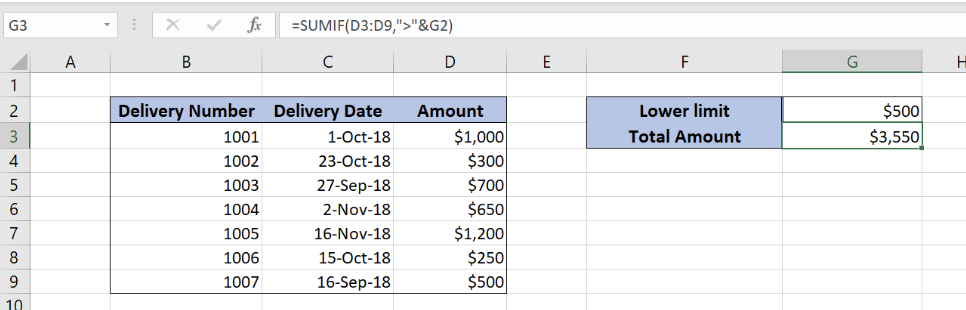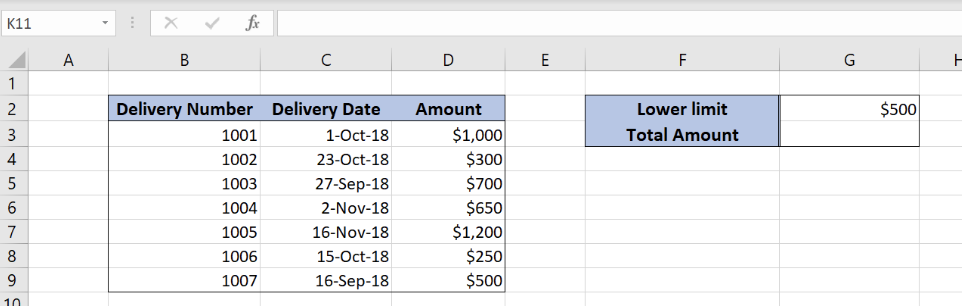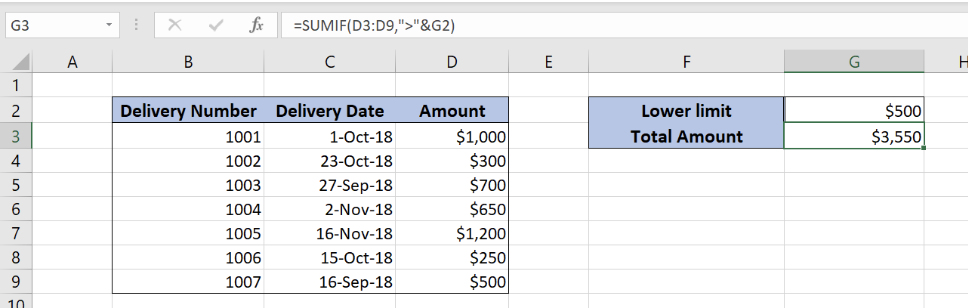Get instant live expert help with Excel or Google Sheets“My Excelchat expert helped me in less than 20 minutes, saving me what would have been 5 hours of work!”

#### Post your problem and you’ll get expert help in seconds.

Your message must be at least 40 characters
Our professional experts are available now. Your privacy is guaranteed.

# Sum if greater than

Excel allows us to sum all values from a table that are greater than selected value by using the SUMIF function. This step by step tutorial will assist all levels of Excel users in summing values from the table with a certain condition.Figure 1. The final result of the SUMIF function

## Syntax of the SUMIF formula

`=SUMIF(range, criteria, [sum_range])`

The parameters of the SUMIF function are:

• range – a range of cells which we want to evaluate
• criteria – criteria for summing data
• [sum_range] – a range of cells that we want to sum. This parameter is not mandatory, so if it’s omitted, a range will be summed.

## Setting up Our Data for the SUMIF Function

Our table consists of 3 columns: “Delivery Number” (column B), “Delivery Date” (column C) and “Amount” (column D). In cell G2, we specify a lower limit, while in cell G3 we want to get a sum of all amounts greater than the lower limit.Figure 2. Data that we will use in the SUMIF example

## Sum Amount greater than the Condition

We want to sum all amounts from column D that are greater than the lower limit (\$500).

The formula looks like:

`=SUMIF(D3:D9,">"&G2)`

The range is D3:D9, while the criteria is “>”&G2.

To apply the SUMIFS function, we need to follow these steps:

• Select cell G3 and click on it
• Insert the formula: `=SUMIF(D3:D9,">"&G2)`
• Press enterFigure 3. Using the SUMIF function to sum values greater than the limit

We see in this example that the formula sums all the amounts that are greater than \$500. As you can see, rows 3(\$1,000), 5 (\$700), 6 (\$650) and 7(\$1,200) meet both conditions, so corresponding amounts are summed. Finally, the sum in the cell G3 is \$3,550.

Most of the time, the problem you will need to solve will be more complex than a simple application of a formula or function. If you want to save hours of research and frustration, try our live Excelchat service! Our Excel Experts are available 24/7 to answer any Excel question you may have. We guarantee a connection within 30 seconds and a customized solution within 20 minutes.

### Did this post not answer your question? Get a solution from connecting with the expert.Another blog reader asked this question today on Excelchat:
Solution examplesamending a formula so that there is another criteria and if it does not meet this criteria then we stick with the original criteria
Solved by A. B. in 40 minsI am looking for a formula to SUM column L, if Column A matched the relevant date and column C matches the name
Solved by Z. Y. in 16 minsI am trying to utilize a complicated sumif or sumifs formula that can pull a total amount from a date and a drop down box from another tab.
Solved by E. B. in 15 minsI have a spreadsheet that totals invoices. A bunch of cells at the bottom of the spreadsheet calculates totals based on which department the invoice is charged to. I'd like to add a function where it also considers the date (so if it's an invoice in January, it goes to a January total, February, etc.). But I can't figure out how to write it properly...
Solved by K. J. in 27 minsIf I want to write a formula that states" "If this name shows up K24, and this word shows up in J24 pull this number from M24" I can creat that formula - however I need to drag this down so it pulls from row 24 - 60 - is there way to do this?
Solved by F. D. in 40 mins## Subscribe to Excelchat.coAnother blog reader asked this question today on Excelchat: